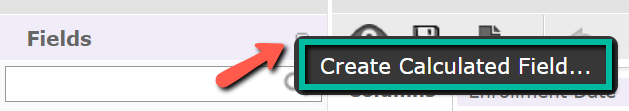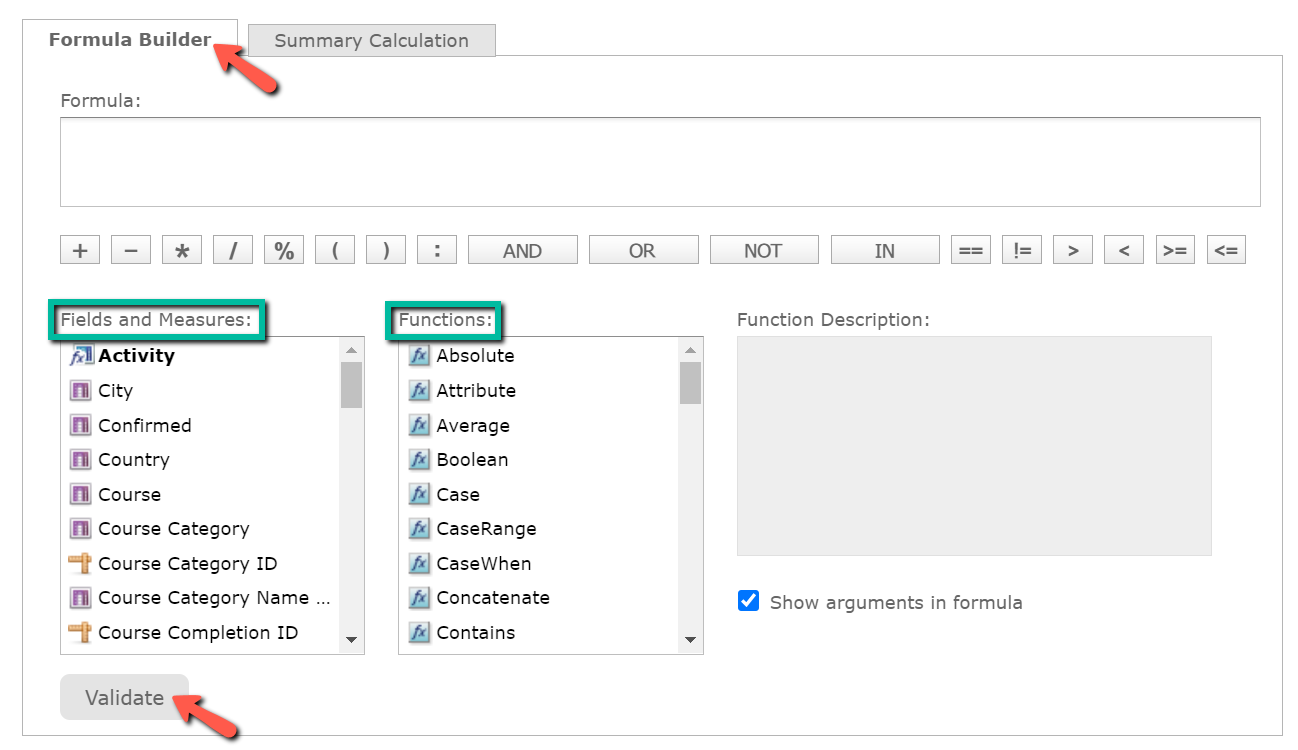# Creating a Calculated Field

## This article focuses on showing how to create calculated fields for Ad Hoc views in Lambda Analytics.

### Calculated Fields

Calculated fields are fields whose values are calculated from a user-defined formula that may include any number of fields, operators, and constants, and are primarily used in data sources or Ad Hoc views. For data sources, a calculated field becomes one of the items to which the data source's security file and locale bundles can apply.

### Creating a Calculated Field

To create a calculated field while creating or editing an Ad Hoc view:

1.  From the Lambda Analytics homepage, click Create under the Ad Hoc view header to create a new Ad Hoc view.

2.  From the Data Selector, select an appropriate data source. Then, click Choose Data to access the Data Chooser.

3.  In the Data Chooser, configure the Fields, Pre-Filters, and Display settings, and click OK to access the Ad Hoc editor.TIP

When creating calculated fields in an Ad Hoc view, it is best to reduce the size of your data set to ensure quick field creation and testing. This can be done through:

• Selecting Sample Data from the Ad Hoc toolbar.
• Creating one or more filters to narrow down your data.
• Limiting the number of fields and measures that you add to your test reports, which will restrict the number of summary levels to one or two.

4.  In the Ad Hoc editor, navigate to the Data Selection panel and hover over the Detail Selector icon at the upper-right corner of the Fields section. Select Create Calculated Field from the context menu to access the New Calculated Field dialog box.5.  Enter an appropriate name for your calculated field in the Field Name field.

6.  Create your formula in the Formula Builder.

This can be done through selecting formulas from the Functions section, and selecting your desired fields from the Fields and Measures section. You can also manually type and create a formula into the Formula Builder.

Click here for a reference guide that lists all the calculated field functions.

7.  Click Validate to test whether or not your formula works.

8.  Once you are complete, click Create Field. The calculated field will then appear at the bottom of the list of available fields. A special Calculated Field icon indicates it is a calculated field.#### Example: Creating a Calculated Field to Convert Grade Percentages

This example will demonstrate how to create a calculated field that shows course results as either Pass or Fail. This can be done through using an IF statement.

An IF statement returns the first value if the condition is true, and the second value if the condition is false.

To create a 'Pass/Fail' calculated field:

1.  In the Ad Hoc editor, navigate to the Data Selection panel and hover over the Detail Selector icon at the upper-right corner of the Fields section. Select Create Calculated Field from the context menu to access the New Calculated Field dialog box.

2.  Enter "Pass/Fail" as your field name in the Field Name field.

3.  Paste the following formula into the Formula Builder:

IF("Course Final Grade" >= 60, 'PASS', 'FAIL')

4.  Click Create Field to return to the Ad Hoc editor. The calculated field will then appear at the bottom of the list of available fields.

This same logic can also be applied to create a calculated field that shows numeric grades as letter grades. Since this requires more conditions, it uses a CaseWhen statement instead:

CaseWhen("Course Final Grade" <= 50, 'F', "Course Final Grade" <= 60, 'D', "Course Final Grade" <= 70, 'C', "Course Final Grade" <= 80, 'B', "Course Final Grade" > 80, 'A')

### Calculated Field Syntax

When creating a calculated field, you will need to use the following syntax when creating an expression:

Text String: To reference a text string, use single quotes ('), e.g. 'Text String.'

Field Label: To reference a field label, use double quotes ("), e.g. "Ad Hoc Label."

Date Constants: To reference date constants, indicate the date type as part of the syntax.

To reference a date without time, data (e.g. yyyy-dd-mm), use "d" followed by single quotes (').

To reference a date with day and time data (e.g. yyyy-dd-mm hh:mm:ss), use "ts" followed by single quotes ('), e.g. "ts'2020-14-12 08:00:00". If you use "ts" and enter the date information only, the time is then automatically set to "00:00:00."

To reference a date with time data only (hh:mm:ss), use "t" followed by single quotes (').

To reference a date field label, use double quotes ("), e.g. "Ad Hoc Date Field Label."NOTES

The following are reserved words and cannot be used as field names: AND, And, and, IN, In, in, NOT, Not, not, OR, Or, or. Names containing these strings, such as "Not Available," can be used.

When dates are used in comparisons or the IF function, they must be the same type (date only, date/time, or time only). Make sure to use the correct modifier (d, ts, t) when using date constants in comparisons.

Boolean Expression: Any expression that takes on Boolean values, including the label of a Boolean field or measure, a Boolean calculation, or a Boolean value. You can create a Boolean expression using the following comparison operators: ==, !=, >, >=, <, <=, in.

Functions that return Boolean values: "StartsWith," "EndsWith," "IsNull," "Contains."

Functions that return logical functions: "AND," "OR," "NOT."

Date Expression: Any type of date or timestamp values, including the label of a date field or measure, or a calculation that returns dates.

Date Time Expression: Date expressions that contain time values, including the label of a date field or measure, or a calculation that returns dates. These values are also known as timestamp values.

Expression: Any valid date, date-time, numeric, or string expression.

Numeric Expression: Numeric values, including the label of a numeric field or measure, or a calculation that returns numbers.

Text Expression: Text values, including the label of a text field or measure, or a text string.

Level: For aggregate functions, specifies the set of values used to compute the calculation.

Possible values include: "Current" (not available for "PercentOf"), "ColumnGroup," "ColumnTotal," "RowGroup," "RowTotal," and "Total."

### Summary Calculations

Summary calculations are aggregate functions used for sub-totals and totals. Once you have entered a formula into the formula builder, the Ad Hoc editor will then create a default summary calculation based on the type of formula that you have created.

#### Default Summary Calculations

 Datatype Default Summary Calculation Description Aggregate AggregateFormula For a calculated field that uses an aggregate function, uses the same aggregate formula as the summary. Boolean CountAll Displays the number of values in the set. Combined None For a calculated field that combines an aggregate function with a non-aggregate function, the summary calculation is null. Date CountAll Displays the total number of values in the set. Numeric Sum Displays the sum of all values in the set. String CountAll Displays the number of values in the set.

To create a custom summary calculation for your calculated field:

1.  After creating your formula on the Formula Builder tab, navigate to the Summary Calculation tab.

2.  Select Custom from the Calculation menu.

3.  Create your custom summary calculation and enter it into the Custom Calculation box. This can be done through selecting an appropriate function from the Functions list, or through manually typing in a formula.

4.  Click Validate to test whether or not your formula works.

5.  Once you are complete, click Create Field.

### Aggregate Functions

Aggregate functions in calculated fields perform a calculation based on groups of rows, rather than on single rows. In many cases, aggregate functions in the Ad Hoc editor are analogous to SQL functions that can be used with the GROUP BY clause in a SELECT statement.

The aggregate functions are as follows:

 Average CountAll MedianMin Range StdDevP WeightedAverage CountDistinctMax Mode PercentOf StdDevsSum

Aggregate functions already operate on groups, so their use is restricted in the following ways:

• You can only use aggregate functions in calculated measures, and aggregates should not be used to create non-measure fields.
• You cannot add an aggregate function to a group.
• You should not use an aggregate function as a filter.
• Only "AggregateFormula," "Custom," or "None," are supported as summary calculations for aggregate functions.

"Custom" only appears in the Change Summary context menu if you have defined a custom function in the Create Calculated Field dialog box.

### Operators

Ad Hoc views support the following operators in calculated fields. Operators are evaluated in the order they are shown in the table:

 Operator Syntax Description multiply, divide i * j / k Arithmetic operators for numeric types. percent i % j Calculates i as a percent of j; numeric types only. add, subtract i + j - k Arithmetic operators for numeric types only. equal i == j Comparison operators for string, numeric, and date types. not equal i != j less than i < j Comparison operators for numeric and date types only. less than or equal i <= j greater than i > j greater than or equal i >= j IN set i IN ('apples,' 'oranges') Sets can be of any type. IN range i IN ( j:k ) Ranges must be numeric or date types. NOT NOT ( i ) Boolean operators. Parentheses are required for NOT. AND i AND j AND k OR i OR j OR k parentheses () Used for grouping.